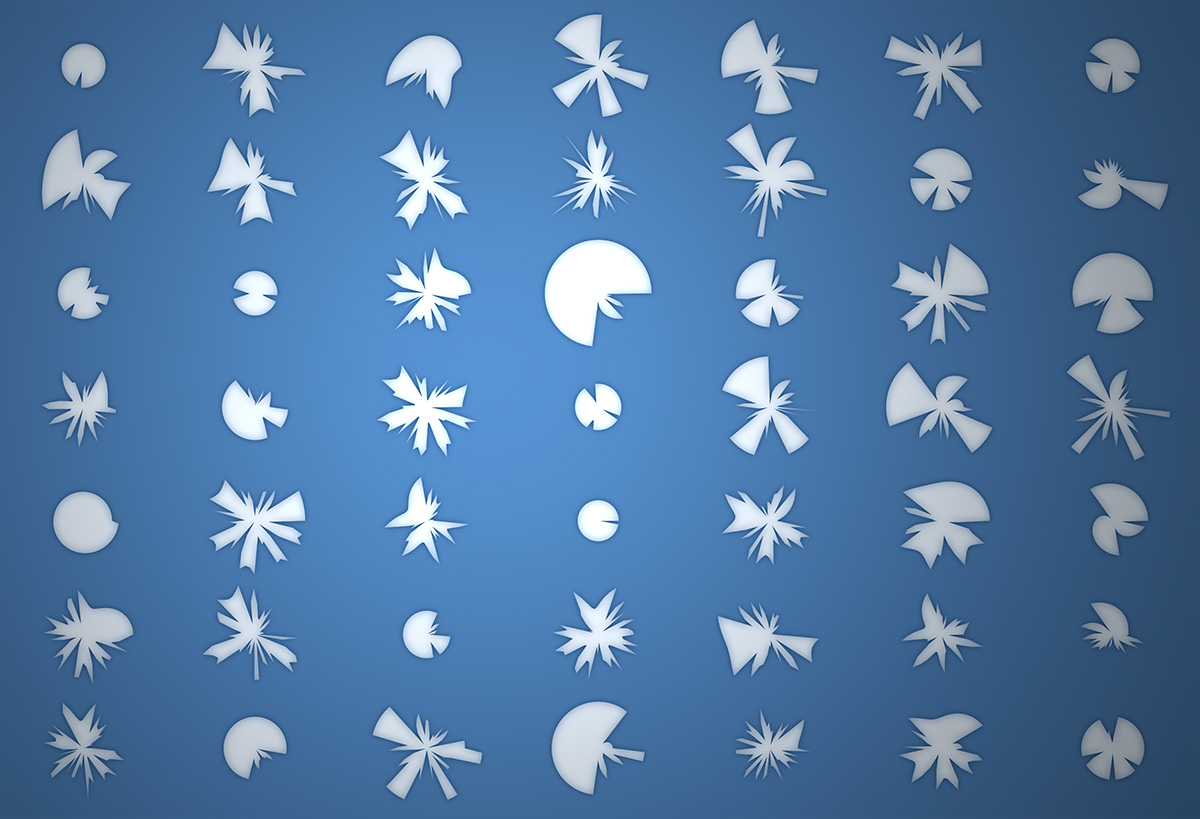# Hermit Crab Curve

#### (or Shard Curve)

by Zevan Rosser

The Shard or Hermit Crab Curve is an equation in polar coordinates with the following form:

$$r = s \sqrt{(a^\pi) \ \text{rem} \sin(d \theta^2 a + o)}$$

Where $a$ and $d$ are paramters that control the detail of the curve. $o$ is a rotation and offset value for the angle and $s$ is a scalar. Note the use of $\textbf{rem}$. The resulting curve is much less interesting if a standard modulo is used in place of a remainder.

$$a = 1.2697968069666183 \\ d = 0.16792929292929293 \\ o = 3.957768393782207 \\ s = 10.7$$

The above variable values were found using this interactive version of the equation.

## $\theta$ Adjustments

In the first plot we step $\theta$ from $0$ to $2\pi$ at an interval of $0.1$. This makes for a simplified angular rendering of the plot. Changing the step value to $0.01$ increases the plots detail:

The result is quite different - from a purely aesthetic view I prefer the lower step resolution (at least in the case of this set of variable values).

## Circular Formations

With $a$ values less than $1$, this equation produces curves with a noticable radius. This is where the remainder aspect of the equation comes into play - capping the values of the pedal curves that would arise without the $(a^\pi \ \text{rem})$ part of the equation.

## Spiked Formations

When $a$ is greater than $1$. We end up with more spike shaped plots:

## Variations

To illustrate the range of types of plots this curve can generate, here is a grid of $49$ plots with randomly varied parameters.